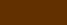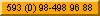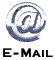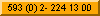How solar photovoltaic electrification works?
How solar photovoltaic electrification works?
CI
Calculation for solar electrification
Calculation for solar electrification PV
ENERGY = POWER  x  HOURS

Wh     =       W      x       h
To calculate an electric solar system, first you have to determine your daily average CONSUMPTION OF ENERGY.
You can realize your calculations of your daily average consumtion of every equipment you want to use, the same way as in the following example, multipying the POWER with the HOURS of usage.
Quantity

A
Equipment

B
Power
W
C
1

Power
W Subtotal
D = (A x C)
Lamps fluores.

15

4

Wh / day
60

60
Hours / day
de usage
E
Energy
Wh
F= (D x E)
15

Total =
1 energysaving lamp for 4 hours
Example A:
The fotovoltaic solar panels capture o transform the sunrays into electric energy. In any case, your daily consumption or the solar system generation is mesured in ENERGY and not only "POWER".

In the following calculations you have to know that ENERGY is your POWER used (multiplied by) certain HOURS of usage.2

1

1
Lamps floures.

Television
15

10

60
4

4

2

Wh / day
120

40

120

280
30

10

60

Total =
2

Lamps fluores.

15

4

Wh / day
120

120
30

Total =
In this example you calculate the daily energy consumtion of 1 flourescent lamp used for 4 hours a day. You multiply (if you want to, in your computer spreadsheet) the quantity of equipment with its power consumption in W (Watts) multiplied with the h hours of usage.
The daily energy consumption in this example is 60 Wh / day.
In this example you can see, that if you conect double of power, your energy consumption also is double.
The daily energy consumption in this example is 120 Wh / day.
Example C:  "Normal Country house"
In this example you can see, the energy consumption of 2 lamps conected for 4 hours is the same as 1 television used for 2 hours. The reason why is, that the enregy consumption of the TV is 4 times bigger than the lamps.
The daily energy consumption in this example is 280 Wh / day.

For this daily energy consumption, you need to install (more or less) one 110 W (Watt) fotovoltaic solar panel, which will cost you aproximately 600 - 900 \$. (more details in the following pages).
2 lamps, 1 radio, 1 television
2 energysaving lamps for 4 hours
Example B:
2

1

1

1
Lamps floures.

Television

Cooler
15

10

60

63
4

4

2

24

Wh / day
120

40

120

1.500

1.780
30

10

60

63

Total =
Example D:  "Bigger Country house"
2 lamps, 1 radio, 1 television, 1 refrigerator
We like to explain here, how to calculate the power of the cooler:
The power of a small cooler (1/4 HP) is aprox. 250 W, but it is conected and disconected in cicles (depends on usage, the temperature inside and outside the cooler, the model, etc.) normally 15 minutes working and 45 minutes in standby, which is the same as an average power of 60 W.
For this daily energy consumption, 1780 Wh/d you need to install (more or less) 5 solar panels 110 W (Watt), which will cost you aproximately 3000 - 4500 \$. (more details in the following pages).
In this example you can see, that the energy consumption of the cooler is 5 times bigger than the rest of consumers, your lamps, radio and TV.
The cooler has got nearly the same POWER as the TV, but the cooler is 24 hours a day conected, meanwhile the TV is used only 2 hours a day.
Calculations for solar electrificationSolar electric systems independent and GRID injection (electrical network). Backup and protection of medical and computer equipment, radio communication, electric fences, cogeneration together with electro-thermal generators, air conditioning, etc.Solar water pumping
Photovoltaic water pump systems that do not require batteries so the return on investment is very fast. These systems work fully automatic.
Quantity

A
Equipment

B
Power
W
C
Power
W Subtotal
D = (A x C)
Hours / day
de usage
E
Energy
Wh
F= (D x E)Quantity

A
Equipment

B
Power
W
C
Power
W Subtotal
D = (A x C)
Hours / day
de usage
E
Energy
Wh
F= (D x E)Quantity

A
Equipment

B
Power
W
C
Power
W Subtotal
D = (A x C)
Hours / day
de usage
E
Energy
Wh
F= (D x E)
Capacitacìon en computacion